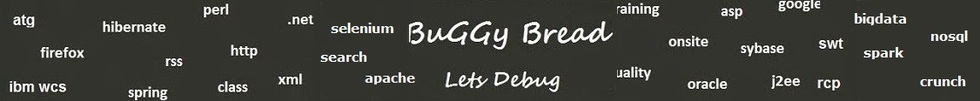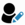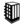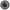Data structure - Interview Questions and Answers for 'Newgen' | Search Java Interview Question - javasearch.buggybread.com# Search Interview Questions3235 questions in repository.There are more than 200 unanswered questions.Have a video suggestion.
Click Correct / Improve and please let us know.
Label / Company      Label / Company / TextSubmit Question

Data structure - Interview Questions and Answers for 'Newgen' - 1 question(s) found - Order By NewestQ1. Write a program for binary tree ?Data Structure2017-09-15 08:00:14Ans. public class BinarySearchTree {
//Represent the node of binary tree
public static class Node {
int data;
Node left;
Node right;
public Node(int data) {
//Assign data to the new node, set left and right children to null

this.data = data;
this.left = null;
this.right = null;
}
}
//Represent the root of binary tree

public Node root;
public BinarySearchTree() {
root = null;
}

//factorial() will calculate the factorial of given number

public int factorial(int num) {
int fact = 1;
if (num == 0) return 1;
else {
while (num > 1) {
fact = fact * num;
num--;
}
return fact;
}
}

//numOfBST() will calculate the total number of possible BST by calculating Catalan Number for given key

public int numOfBST(int key) {
int catalanNumber = factorial(2 * key) / (factorial(key 1) * factorial(key));
return catalanNumber;
}
public static void main(String[] args) {
BinarySearchTree bt = new BinarySearchTree();

//Display total number of possible binary search tree with key 5

System.out.println("Total number of possible Binary Search Trees with given key: "
bt.numOfBST(5));
}
}Help us improve. Please let us know the company, where you were asked this question :LikeDiscussCorrect / ImproveNewGenRelated QuestionsWhat is a binary tree ?Write code for Binary tree traversal ?Flatten a Binary Tree to Linked ListWrite code to check if a Binary tree is symmetricalWrite program to balance a binary treeGiven a sorted array, write an algorithm to create a binary tree.Write a Program to find depth of node binary tree ?

Subscribe to Java News and Posts. Get latest updates and posts on Java from Buggybread.com